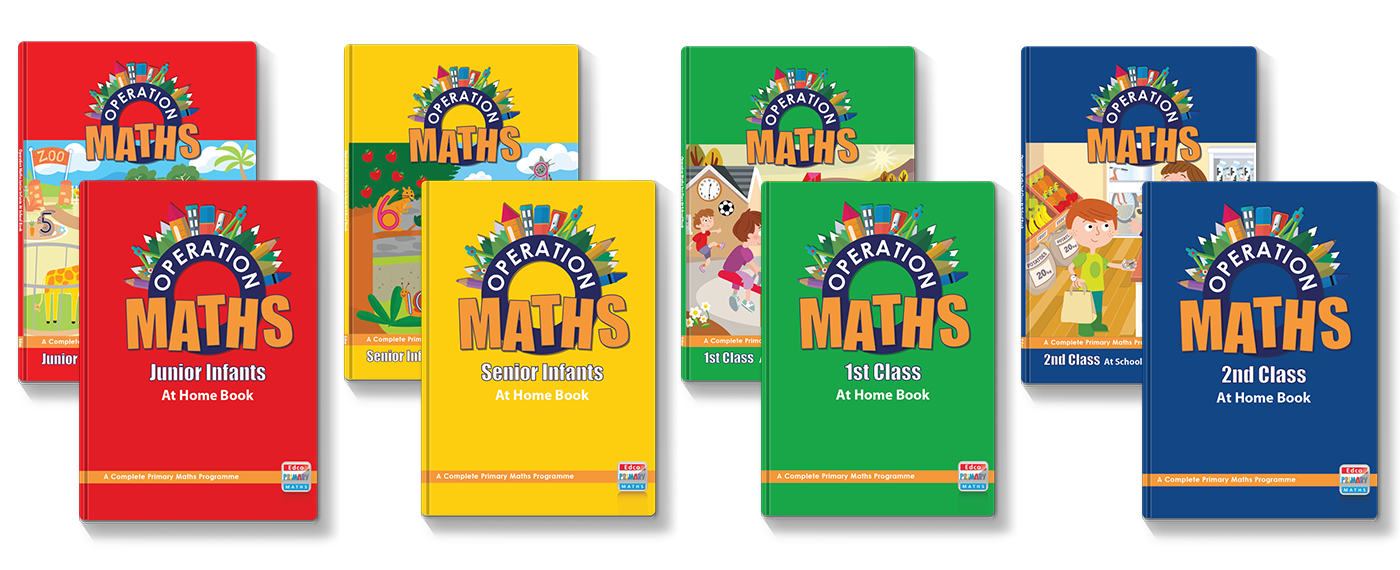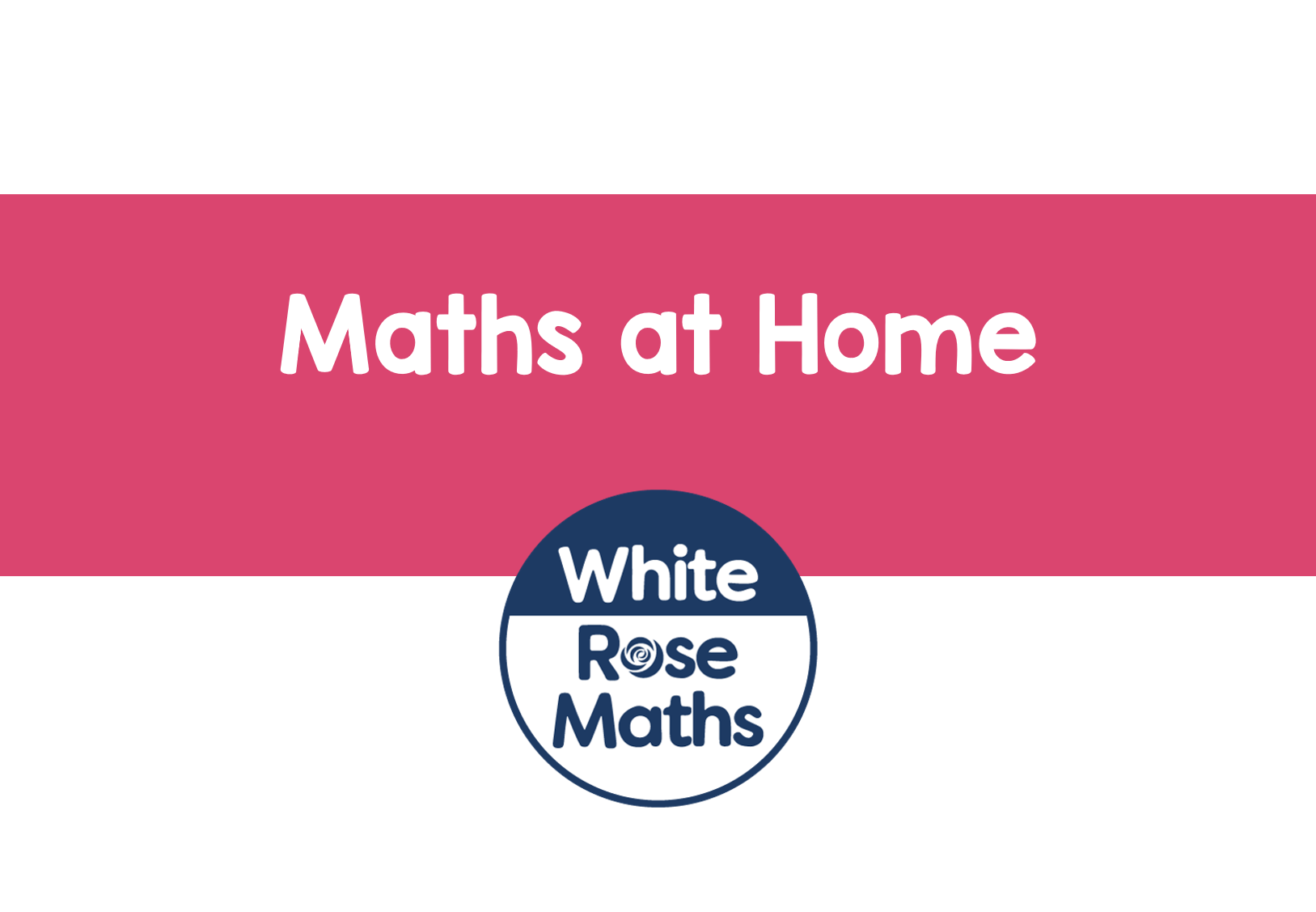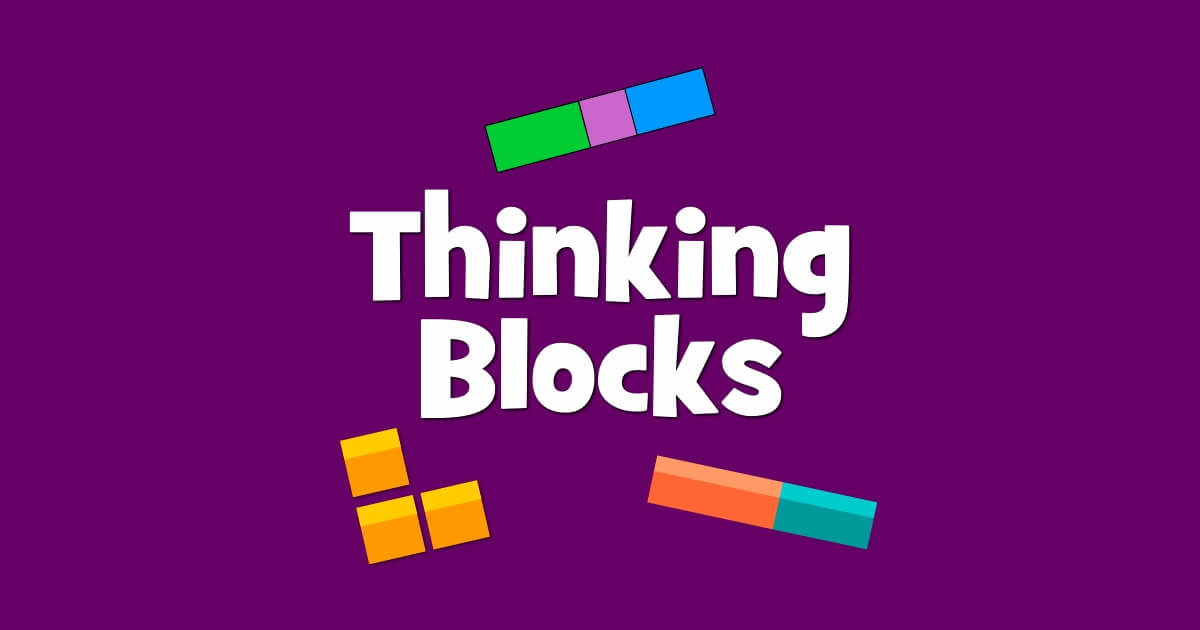• ###### -

Category : Uncategorized

Dear Family, given below is a brief guide to understanding the topic of addition and subtraction, as well as some practical suggestions as to how you might support your children’s understanding at home. Also below, are a series of links to digital resources that will help both the children, and you, learn more about addition and subtraction. The digital resources are organised according to approximate class level:You can also find class specific tips at the back of your child’s Operation Maths At Home book, for infants to second class, and in the Operation Maths Dear Family letters for third to sixth class.

Of all the topics in primary maths, this is probably THE one with which the grown-ups feel most familiar…sure isn’t all just about either adding or subtracting (take away), plus (+) or minus (-), total or difference?

In some ways, it is, and in other ways, it’s not.

As the song goes, ‘it’s not what you do, it’s the way that you do it’ and the same is true for addition and subtraction at primary level; it’s not so much about getting a correct answer (although accuracy is always to be valued in maths) as understanding and visualising what is happening to the amounts/numbers, how they are changing, why they are changing and how the same calculation can be done in many different ways. This is often referred to as developing a child’s number sense.

In Operation Maths, the children are always encouraged to use materials and pictures, and to explore different strategies (ways) to get their answers. They are also encouraged to compare their strategies with the strategies of others, and to explain or justify why one way might be more efficient (faster and/or simpler) than another way.

In particular, the children are encouraged to refer to some key, benchmark facts, that, in turn, can be used to work out other facts. These include doubles (e.g. 4 + 4, 9 + 9 etc), near doubles (e.g. 4 + 5, 9 + 10 etc) and number bonds for 10 (e.g. 4 + 6, 9 + 1 etc). These benchmark facts (or friendly facts), combined with other strategies, such as count on (for adding smaller numbers eg 0, 1, 2, and 3), adding 10 and make a ten, ensures that the child develops both understanding of, and fluency with, the basic addition and subtraction facts, which in turn cam be applied to more complex situations later, such as bigger numbers or fractions and decimals. Pictured below are examples of some useful thinking strategies for the basic addition and subtraction facts.

While addition and subtraction are separate operations, they are also closely very closely related, being the inverse (or opposite) of each other e.g. 10 + 20 = 30, 30 – 20 = 10, 30 – 10 = 20. This is another strategy that the children can use i.e. think addition to do subtraction, or vice versa. Beginning in first and second classes, the children will explore addition and subtraction as being the inverse of each other, which will progress towards using the inverse (opposite) operation to check their calculations.

Therefore, nowadays, there is less emphasis on just learning off “tables”, (i.e. memorising answers) and more emphasis on thinking strategies to use what they know, to solve what they do not know.

#### Practical Suggestions for Supporting Children

• Involve your child in any adding and subtracting that happens at home, or when out and about, e.g. buying tickets for the cinema, buying items of clothes, etc.
• Encourage your child to use strategies when adding and subtracting and/or to explain their strategies. Ask your child to explain how they know, or worked out the answer.
• “I don’t believe you…prove it!” When your child arrives at an answer, ask him/her to show you how they got an answer, to prove that that is the answer, and encourage them to think of other ways to arrive at the same answer. Are there ways that might be more efficient (better) than others?
• Encourage your child to estimate. Estimation is a very important skill; this involves quickly getting a rough or ‘ballpark’ idea of the answer, e.g. €31 and €52 is roughly €80. Encourage your child to quickly estimate totals and differences, e.g. How much, roughly, is that going to cost? Do we have enough money? About how much will we have left?

#### Digital Resources for Junior and Senior InfantsHappy Numbers Pre-Kindergarten: Work through the activities from Module 5, addition and subtraction stories. Alternatively, go to Kindergarten, Module 4, Number Pairs, Addition and Subtraction to 10.I Know it! – Addition & Subtraction: Scroll to the addition and subtraction sections to do any of the activities.Splash Learn – Addition and Subtraction Games: An assortment of games organised according to US grade levels; junior and senior infants should choose among the games for kindergarten level.Addition practice games and Subtraction practice games from ixl.com. You can do a number of free quizzes each day without having a subscription. (Please note that the class levels given do not always align accurately with the content of the Irish Primary Curriculum)

#### Digital Resources for First and Second ClassesHappy Numbers First Grade: First class pupils could work through the activities from Modules 1, 2, 4 and 5. Second class could go to the activities for Second Grade

and work through the activities from Modules 1 and 4.White Rose Addition & Subtraction: a series of lessons on addition and subtraction. These lessons could be followed up with other addition and subtraction lessons in year 1 or year 2Khan Academy Addition and Subtraction: Watch the videos and then answer the practice questions. You can also register for a free Khan Academy account to record your progress and explore other areas of First Grade Maths. Second classes could also look at Addition and Subtraction within 100 in Second Grade MathsHit the Button: a great site for practicing quick-fire Number Bonds (up to 10, 20 or 100) and DoublesThinking Blocks Junior: A simpler version of the original Thinking Blocks game for addition and subtraction, this is an ideal way for first or second classes to start using bar models to model word problems and computation.That Quiz – Arithmetic: Use this quiz to practice different types of addition and subtraction calculations.I Know it! – Addition & Subtraction: Scroll to the addition and subtraction sections to do any of the activities. If too simple, go to Grade Two, to try the activities involving addition and subtraction of 2-digit numbers only.Splash Learn – Addition and Subtraction Games: An assortment of games organised according to US grade levels; start with the games for first grade level. If too simple, go to the Grade Two games.Addition practice games and Subtraction practice games from ixl.com. You can do a number of free quizzes each day without having a subscription. (Please note that the class levels given do not always align accurately with the content of the Irish Primary Curriculum)

#### Digital Resources for Third to Sixth ClassesHappy Numbers Third Grade: Third class pupils could work through the activities from Module 2, Topics C and D. Fourth class pupils, and above,  could go to the activities for Fourth Grade and work through the activities in Module 1.White Rose Addition & Subtraction: a series of lessons on addition and subtraction. These lessons could be followed up with other addition and subtraction lessons in year 3, year 4, year 5 and/or year 6Khan Academy Addition and Subtraction (Third Grade): Watch the videos and then answer the practice questions. For something more challenging,  look at Addition and Subtraction in Fourth Grade Maths. You can also register for a free Khan Academy account to record your progress and explore other topics/grades.Hit the Button: a great site for practicing quick-fire Number Bonds (up to 100) and Doubles.Thinking Blocks for Addition and Subtraction: an ideal way to start using bar models, or further practice using bar models, to model word problems and computation.That Quiz – Arithmetic: Use this quiz to practice different types of addition and subtraction calculations. You can use the options on the left hand side to make the types of calculations easier or more difficult.Estimating sums and differences to win chances to take penalties in a shoot out.I Know it! – Addition & Subtraction (Grade three): Scroll down to the addition and subtraction section to do any of the activities. If too simple, go to the addition and subtraction section in Grade Four or Grade Five.Splash Learn – Addition and Subtraction Games: An assortment of games organised according to US grade levels; start with the games for third grade level. If too simple, go to the Grade Four games.Addition practice games and Subtraction practice games from ixl.com. You can do a number of free quizzes each day without having a subscription. (Please note that the class levels given do not always align accurately with the content of the Irish Primary Curriculum)